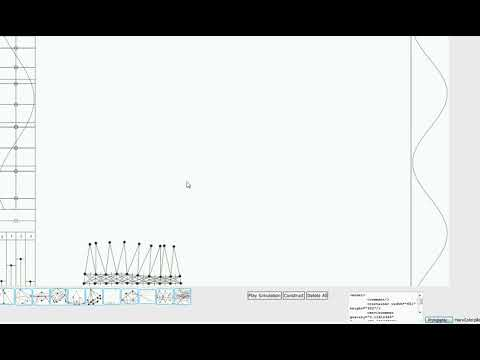## 2D Ripple Algorithm

by Maciej Matyka, Wrocław, 2018-03-13

(play here)

Once upon a time... I saw this on the famous Hugo Elias website (bouncy 2d graphics website). It was around 1998. Now I found it's web archived version of the algorithm and quickly done some re-implementation in javascript.

The basic algorithm works on two 2D tables. You ping-pong the data between tables, so you first run from 1 to 2 and then back from 2 to 1.

The main wave propagation goes like this:

for each particle (x.y) calculate:
map2(x,y) = (map(x-1, y) +
map(x+1, y) +
map(x, y+1) +
map(x, y-1)) /2 - map2(x,y)
map2(x,y) = map2(x,y) * damping

where damping is a number between 0-1. After one step map becomes map2 and vice-versa.
It is worth to add some smoothing as well (simply average each pixel between rendering).

Note: I discovered that using single pixel as the starting point for the wave gives really ugly picture and, thus I put the 3x3 picel each time I want to seed the wave.

Below is the first result of the model implemented in 2018 by me.

The app will be soon available at http://felp.pl.

1.2.